# (1 point) For the circuit below, V, (148 - 173°) V, C 192 2, and R...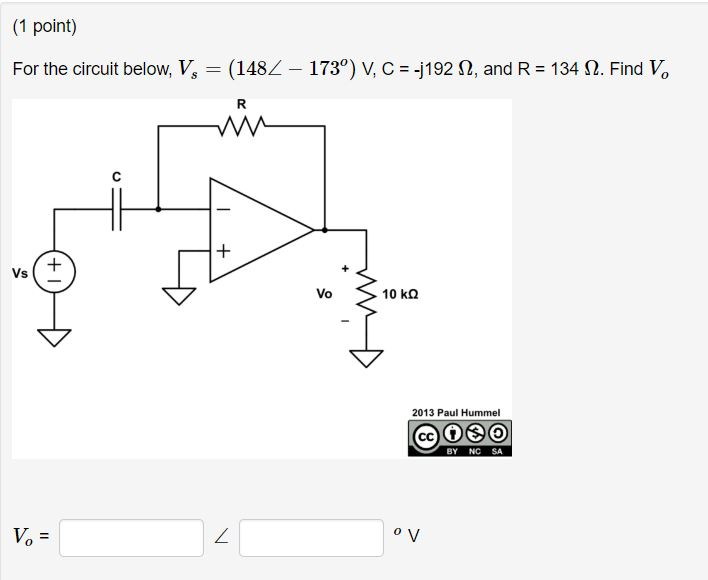(1 point) For the circuit below, V, (148 - 173°) V, C 192 2, and R 134 S2. Find Vo Vs Vo 10 k? 2013 Paul Hummel @OEO BY NC SA 0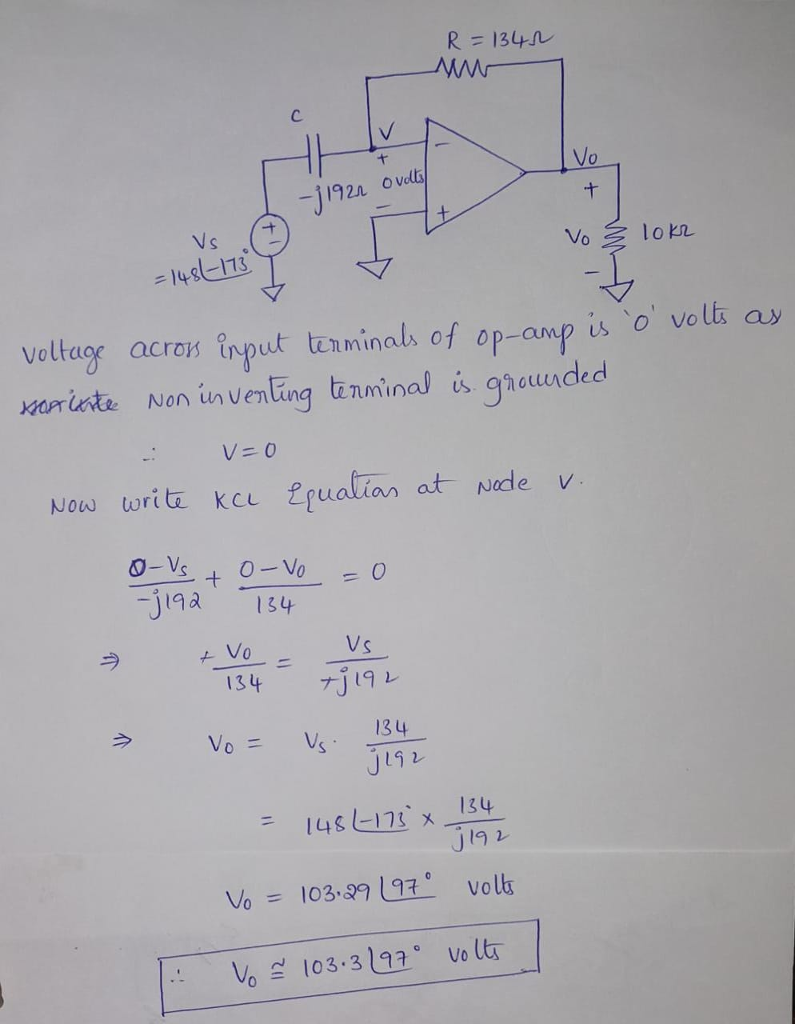#### Earn Coin

Coins can be redeemed for fabulous gifts.

Similar Homework Help Questions
• ### (1 point) For the circuit below, V,--(162434°) ?, C--j1 52 ?, and R-100 ?. Find V,...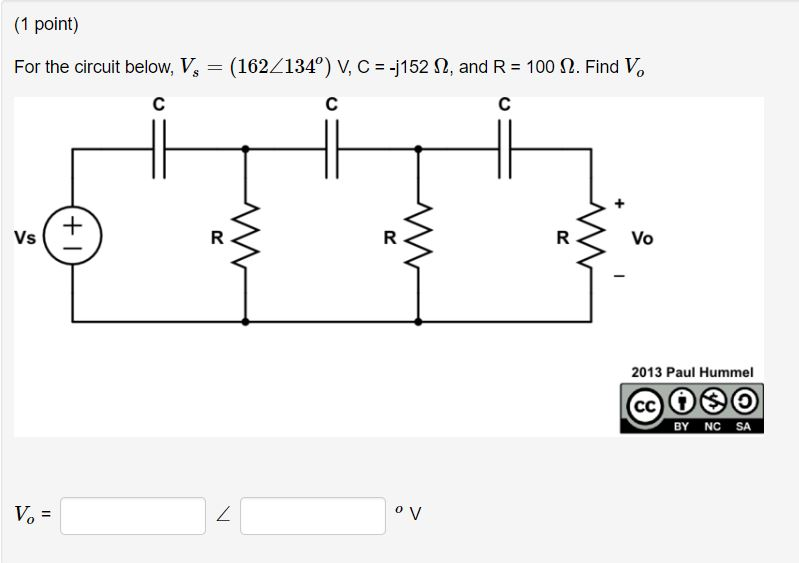(1 point) For the circuit below, V,--(162434°) ?, C--j1 52 ?, and R-100 ?. Find V, Vo 2013 Paul Hummel BY NC SA Vo

• ### (1 point) For the circuit below, V, = (1802 – 29°) V, C = -j170 12,...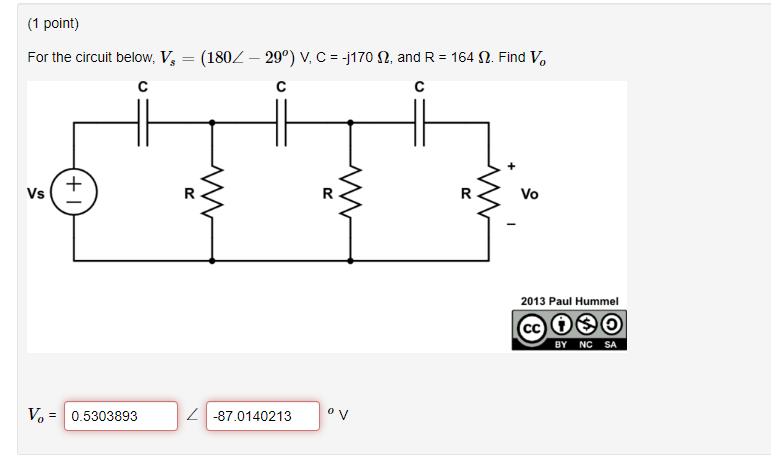(1 point) For the circuit below, V, = (1802 – 29°) V, C = -j170 12, and R = 164 12. Find V. R Vo 2013 Paul Hummel © 000 BY NC SA V = 0.5303893 2 -87.0140213 v

• ### (1 point) In the circuit below, V1 = 8.5 V and 11 = 450 mA. Use...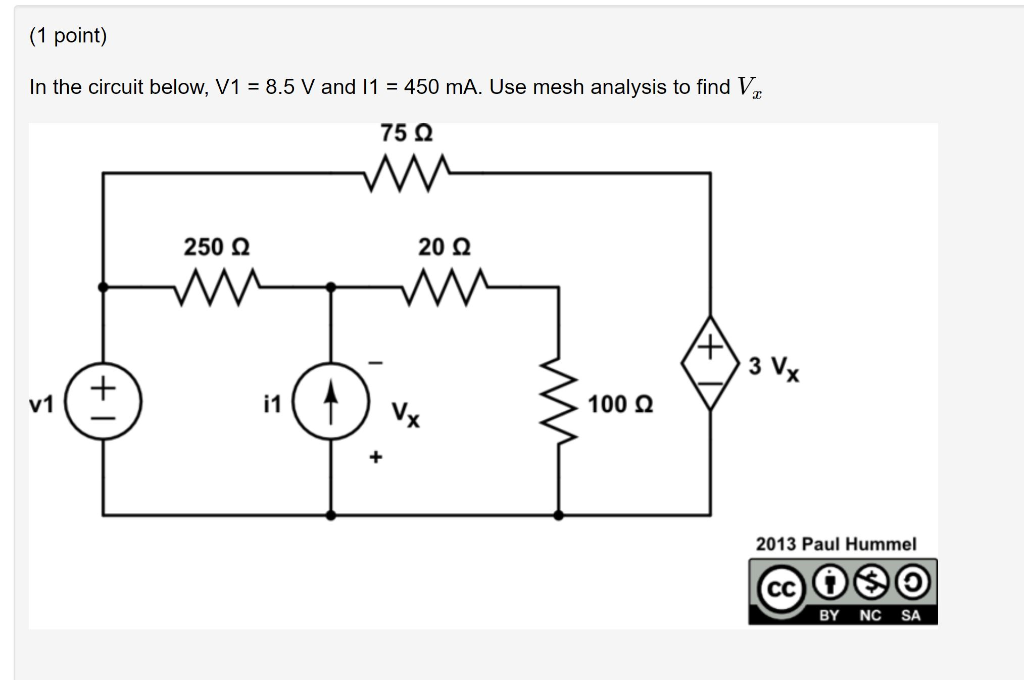(1 point) In the circuit below, V1 = 8.5 V and 11 = 450 mA. Use mesh analysis to find V. 75 22 250 22 20 22 M AAA 100 22 2013 Paul Hummel BY NC SA

• ### (1 point) For the circuit below, V1 = 13 V, V2 = 8 V, V3 =...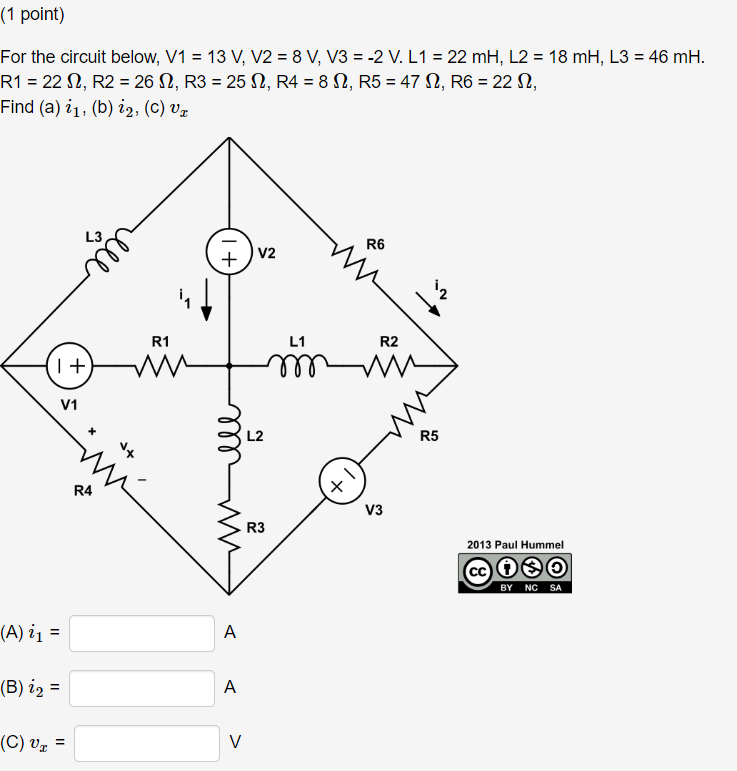(1 point) For the circuit below, V1 = 13 V, V2 = 8 V, V3 = -2 V. L1 = 22 mH, L2 = 18 mH, L3 = 46 mH. R1 = 22 12, R2 = 26 12, R3 = 25 12, R4 = 8 12, R5 = 47 12, R6 = 22 12, Find (a) i1, (b) i2, (C) v. 1+ RG V2 min to-rööviz w ell + V3 Zamo 2013 Paul Hummel BY NC SA (A) 11 =...

• ### (1 point) In the circuit below, R1 = 70 12, R2 = 40 S2, R3 =...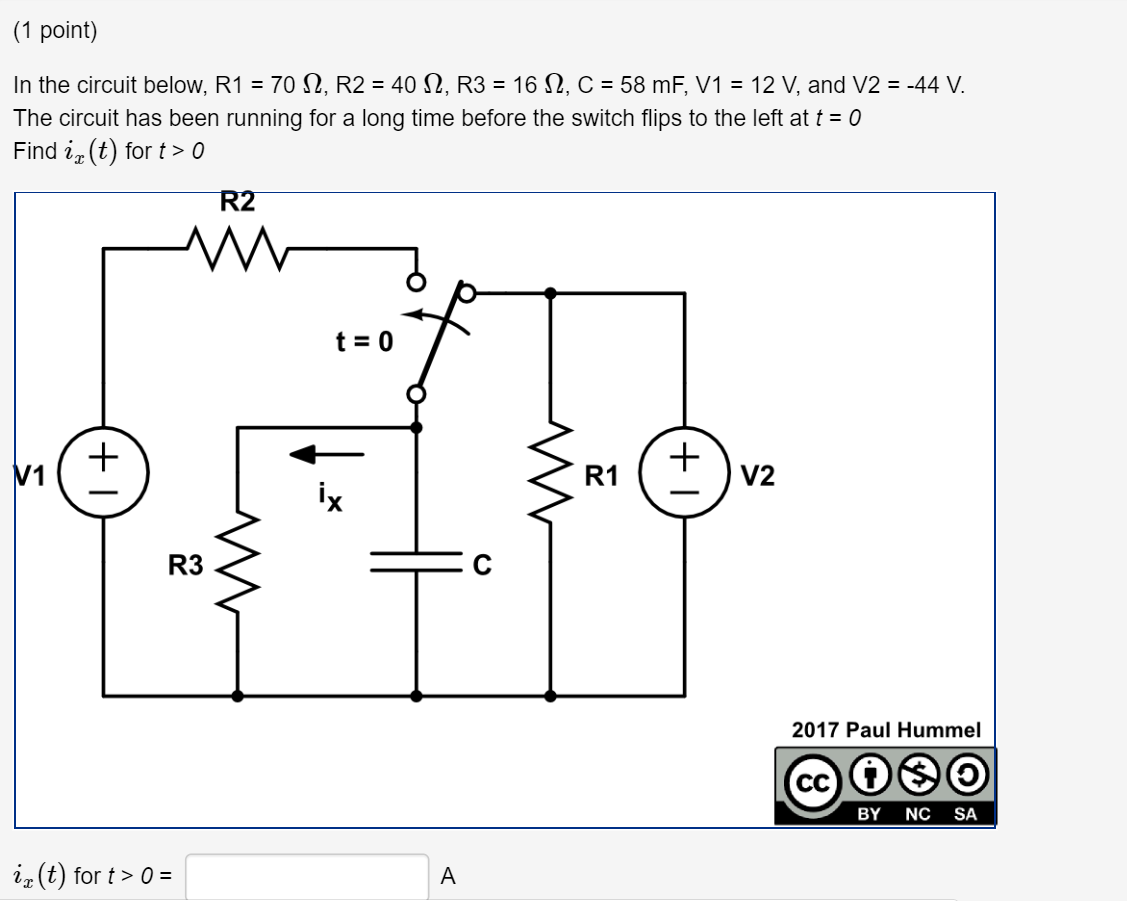(1 point) In the circuit below, R1 = 70 12, R2 = 40 S2, R3 = 16 12, C = 58 mF, V1 = 12 V, and V2 = -44 V. The circuit has been running for a long time before the switch flips to the left at t = 0 Find iz(t) for t > 0 R2 t= 0 R3S 2017 Paul Hummel CCOO BY NC SA iz(t) for t> 0 =

• ### In the circuit below, v1 = -2 V and i1 = -4.5 A. Use mesh analysis...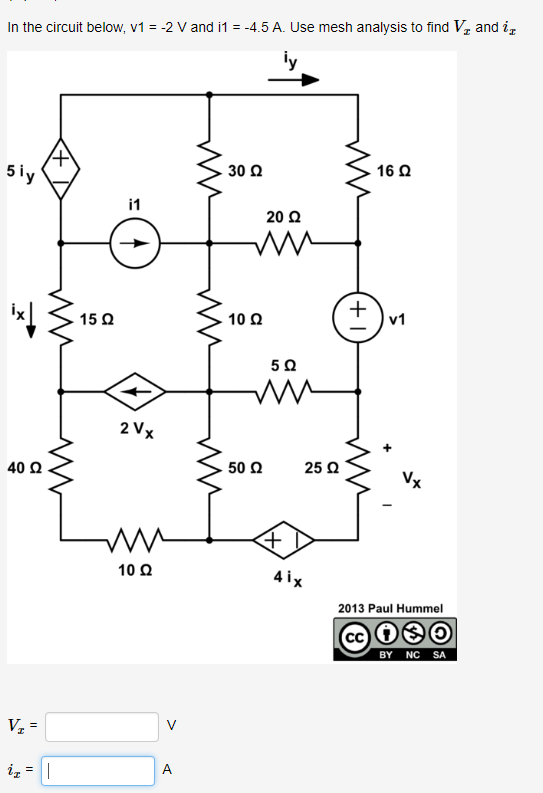In the circuit below, v1 = -2 V and i1 = -4.5 A. Use mesh analysis to find Vx and ix. ne crcat tatalsd 45U mesh 5i 30 Ω 16 Ω i1 20 Ω 10Ω 5Ω 2 Vx 40 Ω 50 Ω 25 Ω V. 10Ω 4 i 2013 Paul Hummel BY NC SA

• ### (1 point) In the circuit below, i1 = -2 A, i2 = 8 A, and v1...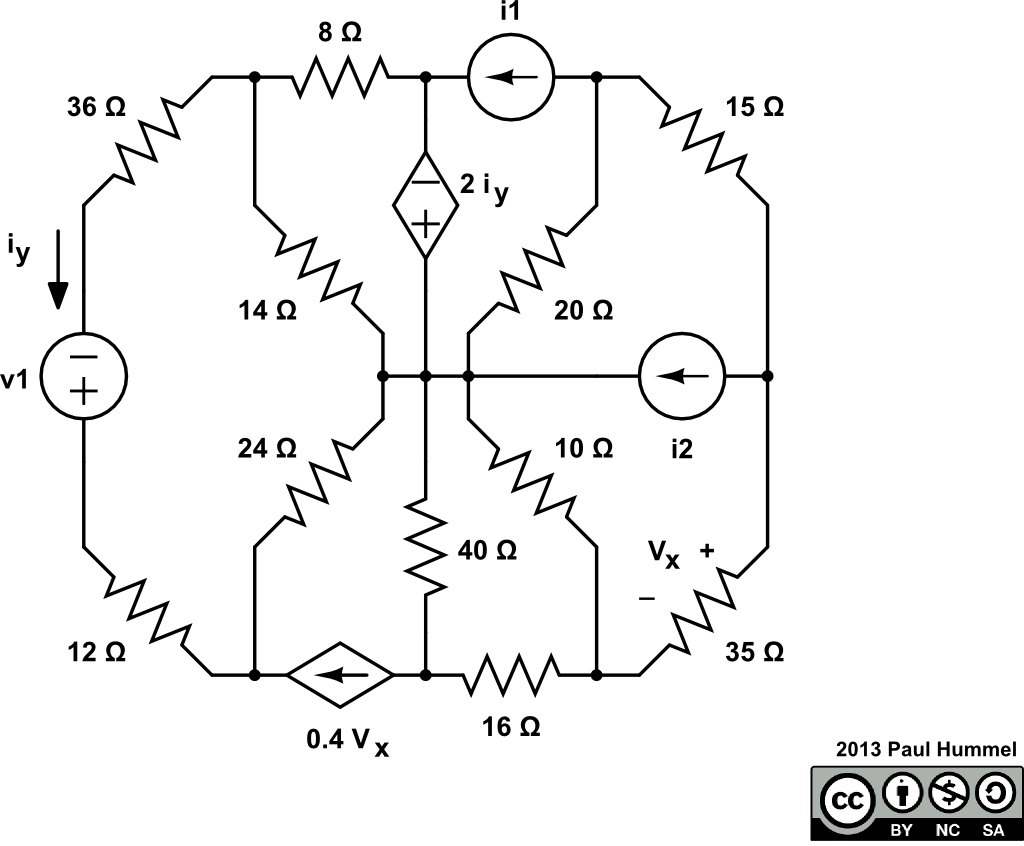(1 point) In the circuit below, i1 = -2 A, i2 = 8 A, and v1 = -2 V. Use nodal analysis to find vx and iy in the circuit below i1 8Ω 15 Ω 36 Ω 2 iy y 14 Ω 20 Ω ν1 24 Ω 10 Ω i2 40 Ω 35 Ω 12 Ω 16 Ω 0.4Vx 2013 Paul Hummel CC BY NC SAΑ

• ### (1 point) Problem 1 In the circuit below: V1 = 180 V, V2 = -150 V,...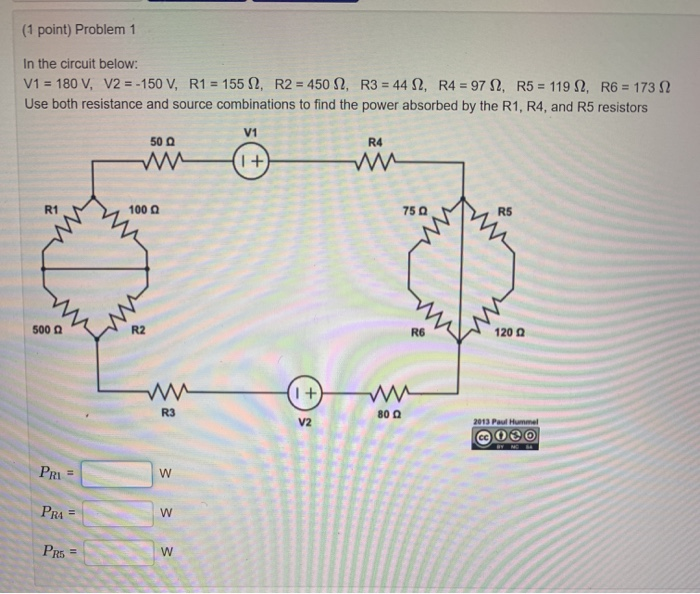(1 point) Problem 1 In the circuit below: V1 = 180 V, V2 = -150 V, R1 = 155 12, R2 = 450 S2, R3 = 44 S2, R4 = 97 12. R5 = 119 12, R6 = 1732 Use both resistance and source combinations to find the power absorbed by the R1, R4, and R5 resistors V1 50 R4 I + R1 100 75 R5 w mi 500 g w R6 120 1 + R3 80 V2 2013 Paul...

• ### (1 point) For a standard capacitor with c=116μF: If vc(t)=4.2+14.8cos2⁡(190t) V, Find: (a) ic(t) (b) The...(1 point) For a standard capacitor with c=116μF: If vc(t)=4.2+14.8cos2⁡(190t) V, Find: (a) ic(t) (b) The maximum stored energy in the capacitor If ic(t)=14.8e−190t mA for t>0 and vc(0)=0, Find: (c) vc(t) for t>0 If ic(t)=14.8e−190t mA for t>0 and vc(0)=4.2 mV, Find: (d) vc(t) for t>0 If the stored energy is w(t)=26e−315tμJ for t>0t>0, Find: (e) ic(t) (a) ic(t) =  mA (b) wmax =  μJ (c) vc(t) =  mV (d) vc(t) =  mV (e) ic(t) =  mA or ic(t) =  mA 2013 Paul Hummel BY...

• ### (1 point) For the equivalent resistances in the circuits below if R = 105 ΩΩ Req...(1 point) For the equivalent resistances in the circuits below if R = 105 ΩΩ Req 50Ω 200 Ω 10Ω (b)Rea 100 Ω 10Ω 20 Ω (c)Rea 30Ω 50 Ω 16 Ω 2013 Paul Hummel